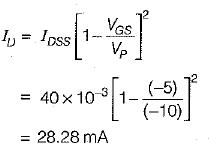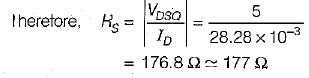Courses

# Basic FET Amplifiers - 1

## 10 Questions MCQ Test Topicwise Question Bank for GATE Electrical Engineering | Basic FET Amplifiers - 1

Description
This mock test of Basic FET Amplifiers - 1 for Electrical Engineering (EE) helps you for every Electrical Engineering (EE) entrance exam. This contains 10 Multiple Choice Questions for Electrical Engineering (EE) Basic FET Amplifiers - 1 (mcq) to study with solutions a complete question bank. The solved questions answers in this Basic FET Amplifiers - 1 quiz give you a good mix of easy questions and tough questions. Electrical Engineering (EE) students definitely take this Basic FET Amplifiers - 1 exercise for a better result in the exam. You can find other Basic FET Amplifiers - 1 extra questions, long questions & short questions for Electrical Engineering (EE) on EduRev as well by searching above.
QUESTION: 1

### When VGS of a JFET changes from -2.4 to-2 V, the drain current changes from 1 mA to 1.4 mA. What is the value of transconductance?

Solution: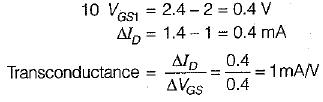QUESTION: 2

### In the CS amplifier shown in figure, if RD = 5 KΩ RG = 10 MΩ, μ = 50, and rd =  35 kΩ.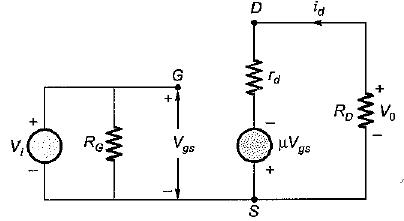The voltage gain (AV) of the amplifier is

Solution:

The output voltage is,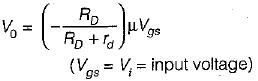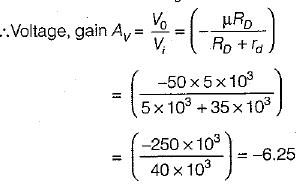QUESTION: 3

### Consider the following statements associated with small signal FET model and A-parameter model of BJT (in CS configuration and CE configuration respectively): 1. Both FET and BJT have a Norton’s output circuit, but the current generated in BJT depends on the input current and not on the input voltage as in FET. 2. There is no feedback from output to input in the FET, whereas a feedback exist in the BJT through the parameter hre. 3. The high input resistance of the FET is replaced by an input resistance of about 1 kΩ for a CE amplifier. Which of the above statements is/are correct?

Solution: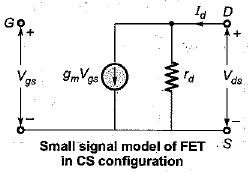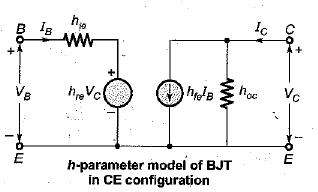By observing the above two modes, we can find that all the statements are correct.

QUESTION: 4

Assertion (A): FET is a much more ideal amplifier than the BJT at low frequencies.
Reason (R): FET has high input impedance and there is no feedback from output to input.

Solution:
QUESTION: 5

Match List-I (Small Signal FET Amplifier Circuits) with List-I I (Voltage Gain) and select the correct answer using the codes given below the lists: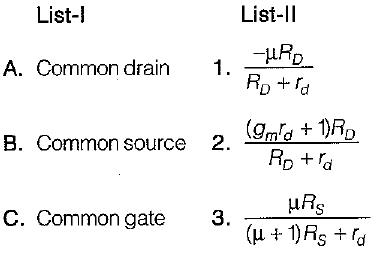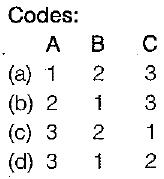Solution:
QUESTION: 6

An N-channe! JFET has IDSS = 8 mA and Vp = -5 V. The minimum values of VDS for pinch- off region and the drain current IDS for VGS= - 2V in the pinch-off region are respectively.

Solution:

The minimum value of VDS for pinch-off to occur for VGS = -2V is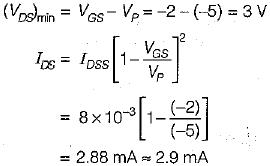QUESTION: 7

Assertion (A): The N-channel MOSFET may turnon prematurely.
Reason (R): The drain resistance of P-channel MOSFET is three times higher than that for an identical N-channei MOSFET

Solution:

The N-channel MOSFET may turn on prematurely due to the positively charged contaminants. Reason is also a correct statement but not the correct explanation of assertion.

QUESTION: 8

Assertion (A): The input noise is less in N-channel JFET than that in P-channel JFET.
Reason (R): The transconductance of N-channel JFET is smaller than that of P-channel JFET.

Solution:

The transconductance of N-channel JFET is more than that of P-channel JFET. Hence, reason is not a correct statement.

QUESTION: 9

A JFET amplifier with a voltage divider biasing circuit, shown in figure has the following parameters:
Vp = -2 V, IDSS = 4 mA, RD = 910 Ω, Rs =3 kΩ,
R1, = 12 MΩ, R2 = 8.75 MΩ and VDD = 24 V.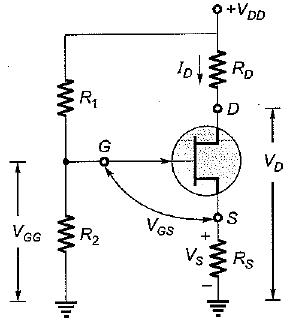The value of the drain current ID at the operating point is

Solution:

We have: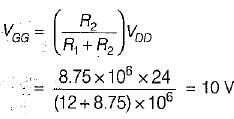We know that,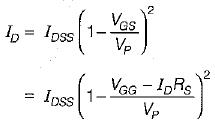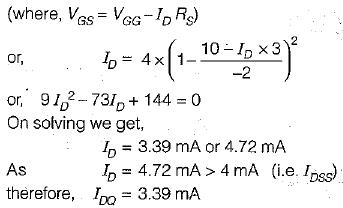QUESTION: 10

The value of source resistance required to self bias an N-channel JFET with IDSS = 40 mA, Vp = -10 V, VGS = -5 V and VDSQ = 5V is

Solution: